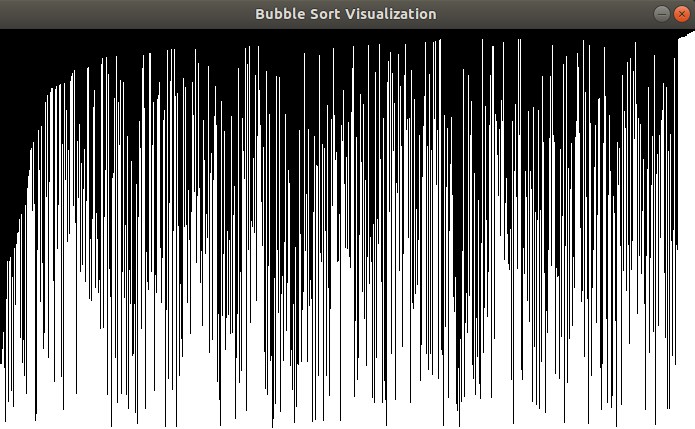# Fun With Python #5: Bubble Sort Visualization

Welcome to “Fun with Python”, part 5. In this part, we will utilize the `pygame `module to visualize bubble sort and get more insights about how it works.

# Theory and Foundations

Every CS student or developer has spent time studying sorting techniques and algorithms and the first and simplest sorting algorithm that gets presented is bubble sort.

Bubble sort is a sorting algorithm that compares adjacent numbers and swaps them if needed. This continues until all the elements are sorted. An example will make things clearer:

We start of with this sequence of numbers and we want to sort them resulting in an ascending sequence:

5, 3, 2, 6, 1, 4

First we compare 5 with 3, with 5 being bigger than 3 so we swap:

3, 5, 2, 6, 1, 4

Next we compare 5 with 2. Again 5 is greater that 2 so we swap:

3, 2, 5, 6, 1, 4

Our next comparison is between 5 and 6. This time, 6 is greater than 5 so we do not swap the two numbers. So we move on and compare 6 with the next number which is 1 and the result is a swap:

3, 2, 5, 1, 6, 4

Finally we compare 6 with 4 and we swap resulting to this sequence:

3, 2, 5, 1, 4, 6

As you can see, the greatest number is now on the last position. If we do this process again, we will swap numbers and 5 will be put to the correct position. If we continue doing this process, we will have the sequence sorted.

From what you have seen, you might already have guessed that performance wise, this is not an optimal algorithm. In fact, its complexity is O(n²) where n is the number of elements that need to be sorted. This makes the algorithm not suitable for practical use cases, where other, more efficient algorithms are being used.

By intuition, one can see that the greatest numbers are put to the end of the sequence in every iteration. Let’s confirm it by visualizing the algorithm. Here, the `pygame` module will be of great assistance.

According to pygame official page:

Pygame is a set of Python modules designed for writing video games. Pygame adds functionality on top of the excellent SDL library. This allows you to create fully featured games and multimedia programs in the python language.

In other words, `pygame` is a module used in Python, in order to create video games, but we are going to use some basic features. Let’s get started.

# Implementation

The first thing we are going to do is create an empty window. We will define the width and the height. We will also give out window a name:

`import randomimport pygamewin_width = 700win_height = 400values = [int(random.random()*win_height) for _ in range(win_width)]pygame.init()win = pygame.display.set_mode((win_width, win_height))pygame.display.set_caption('Bubble Sort Visualization')`

Also, we will create a list of random numbers. We want to create a number for every pixel in the window and we will normalize the values `random.random()` returns so that the whole height of the window gets filled.

Let’s draw our values in the empty window we have:

`run = Truewhile run:    pygame.time.delay(100)    for i in range(0, win_width):        pygame.draw.line(win, (255, 255, 255), (i, win_height),                         (i, values[i]))    pygame.display.update()    for event in pygame.event.get():        if event.type == pygame.QUIT:            run = False`

We create an endless loop, in which for every value in the list, we draw a line, starting from the bottom of the window. The height of each line represents the value of the number being drawn. Also, we check if the user decides to close the window, so that we can exit our program. This is the result we get:

Next, we will implement bubble sort. Implementation is quite simple:

`for i in range(0, len(values) - 1):    for j in range(0, len(values) - i - 1):        if (values[j] < values[j + 1]):            tmp = values[j]            values[j] = values[j + 1]            values[j + 1] = tmp`

Finally, we will insert this code in the loop we created above. After each iteration, we will update our window, with the updated list:

`run = Truedone = Falsewhile run:    pygame.time.delay(100)    if (done == False):        for i in range(0, len(values) - 1):            for j in range(0, len(values) - i - 1):                if (values[j] < values[j + 1]):                    tmp = values[j]                    values[j] = values[j + 1]                    values[j + 1] = tmp            win.fill((0, 0, 0))            for i in range(0, win_width):                pygame.draw.line(                    win, (255, 255, 255),                    (i, win_height),                    (i, values[i])                )            pygame.display.update()        done = True    for event in pygame.event.get():        if event.type == pygame.QUIT:            run = False`

# Conclusion

After the first iterations, you will see the following window:Greatest numbers are moved to the right of the window

As you can see, the greatest numbers start moving to the right of the window. This is exactly how bubble sort works. In every pass, you are trying to bring the greatest number to its correct position (or the minimum if you are sorting ascending). When the sorting finishes, the window will look like this: